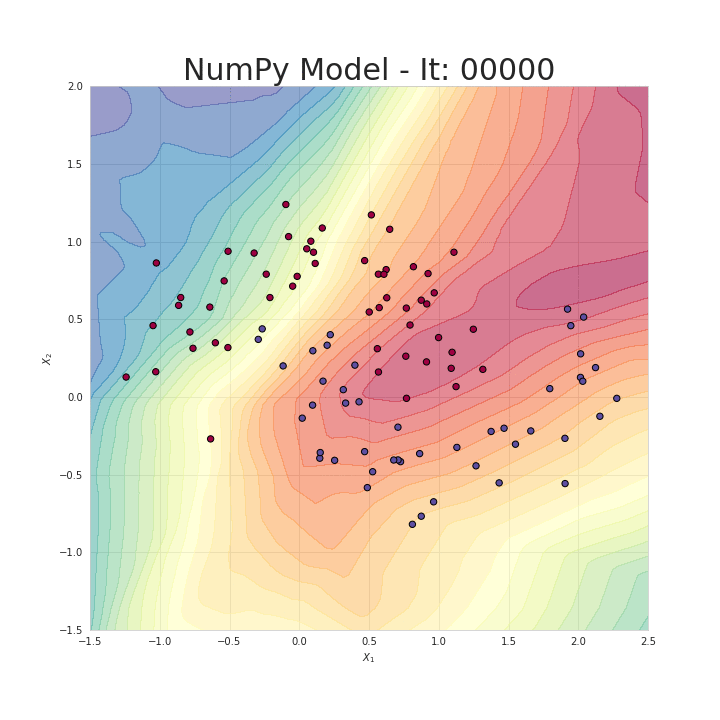# Let’s code a Neural Network in plain NumPy

## Mysteries of Neural Networks Part III

Using high-level frameworks like Keras, TensorFlow or PyTorch allows us to build very complex models quickly. However, it is worth taking the time to look inside and understand underlying concepts. Not so long ago I published an article, explaining — in a simple way — how neural nets work. However, it was highly theoretical post, dedicated primarily to math, which is the source of NN superpower. From the beginning I was planning to follow-up this topic in a more practical way. This time we will try to utilize our knowledge and build a fully operational neural network using only NumPy. Finally, we will also test our model — solve simple classification problem and compare its performance with NN built with Keras.

Note: Obviously, today’s post will consist in large part of code snippets written in Python. I hope, however, that the reading will not be too boring. :) You will also find short comments in places that I consider to be not clear or worthy of attention. As usual, all the source code is available on my GitHub.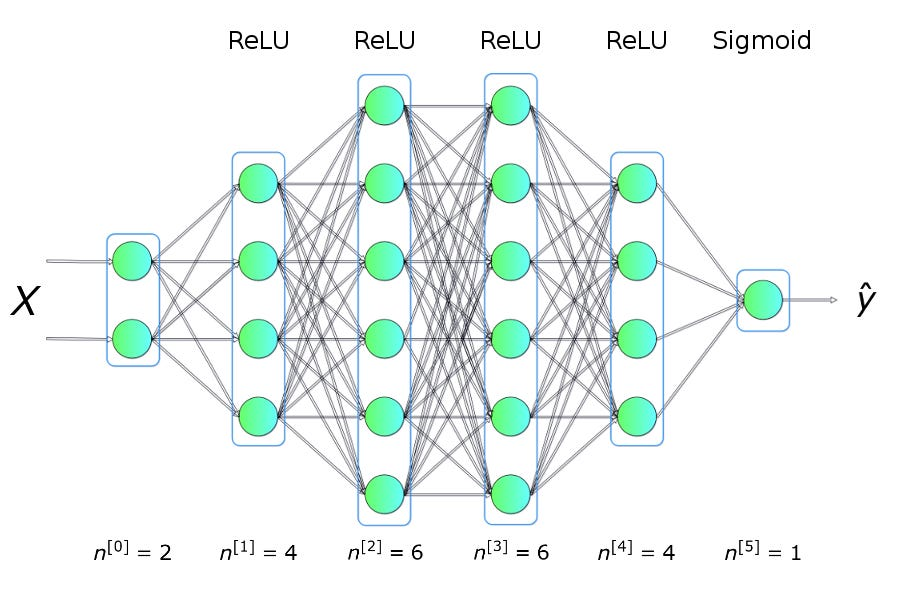Figure 1. Example of dense neural network architecture

#### First things first

Before we start programming, let’s stop for a moment and prepare a basic roadmap. Our goal is to create a program capable of creating a densely connected neural network with the specified architecture (number and size of layers and appropriate activation function). An example of such a network is presented in Figure 1. Above all, we must be able to train our network and make predictions using it.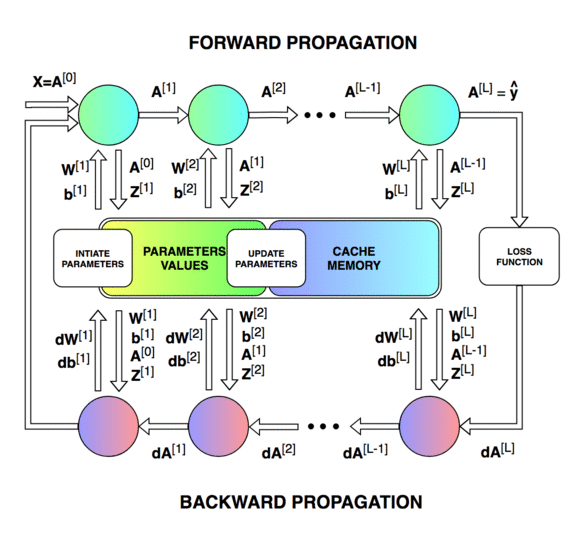Figure 2. Neural network blueprint

Diagram above shows what operations will have to be performed during the training of our NN. It also shows how many parameters we will have to update and read at different stages of a single iteration. Building the right data structure and skillfully managing its state is one of the most difficult parts of our task. Due to time limits, I will not describe in detail the role of each of parameters shown in the figure. I refer all those interested to the first article of this series, where I hope you will find answers to all the questions that bother you.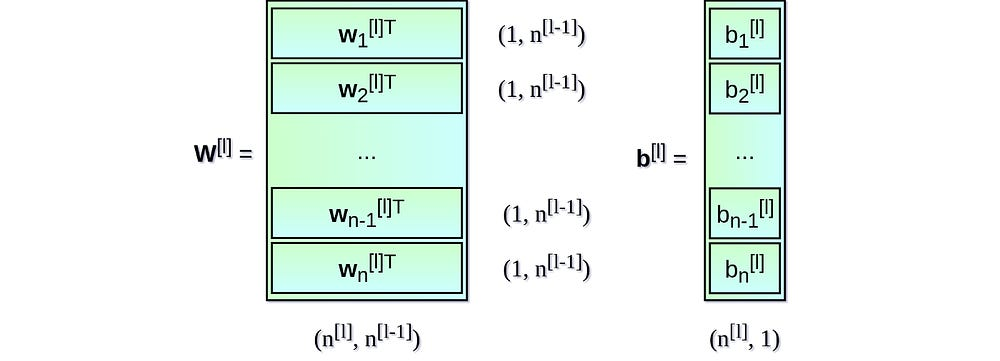Figure 3. Dimensions of weight matrix W and bias vector b for layer l.

#### Initiation of neural network layers

Let’s start by initiating weight matrix W and bias vector b for each layer. In Figure 3. I have prepared a small cheatsheet, which will help us to assign the appropriate dimensions for these coefficients. Superscript [l] denotes the index of the current layer (counted from one) and the value n indicates the number of units in a given layer. I assumed that the information describing the NN architecture will be delivered to our program in the form of list similar to the one presented on Snippet 1. Each item in the list is a dictionary describing the basic parameters of a single network layer: `input_dim` – the size of the signal vector supplied as an input for the layer, `output_dim` – the size of the activation vector obtained at the output of the layer and `activation` – the activation function to be used inside the layer.Snippet 1. A list containing parameters describing a particular neural network. This list corresponds to the NN shown in Figure 1.

If you’re familiar with this subject, you’ve probably already heard a voice in your head speaking with anxious tone: “Hey, hey! Something is wrong! Some of these fields are unnecessary…”. Yeah, your inner voice is right this time. The vector coming out of one layer is also the input for the next one, so in fact it is enough to know the size of only one of those vectors. However, I deliberately decided to use the following notation to keep the objects consistent across all layers and make the code easier to understand for those who encounter these topic for the first time.Snippet 2. The function that initiates the values of the weight matrices and bias vectors.

Let’s finally focus on the main task that we have to accomplish in this part — the initiation of layers parameters. Those who have already looked at the code on Snippet 2 and have some experience with NumPy have noticed that the matrix W and vector b have been filled with small, random numbers. This approach is not accidental. Weights values cannot be initialized with the same number because it leads to breaking symmetry problem. Basically, if all weights are the same, no matter what was the input X, all units in the hidden layer will be the same too. In a way, we got stuck in the initial state without any hope for escape, no matter how long will we train our model and how deep our network is. A linear algebra does not forgive.

The use of small values increases the efficiency of our algorithm during first iterations. Looking at the graph of the sigmoid function, shown in Figure 4, we can see that it becomes almost flat for large values, what has significant effect on the speed of learning of our NN. All in all parameter initiation using small random numbers is simple approach, but it guarantees good enough starting point for our algorithm. Prepared parameters values are stored in a python dictionary with a key that uniquely identifies their parent layer. The dictionary is returned at the end of the function, so we will have access to its contents in the next stages of our algorithm.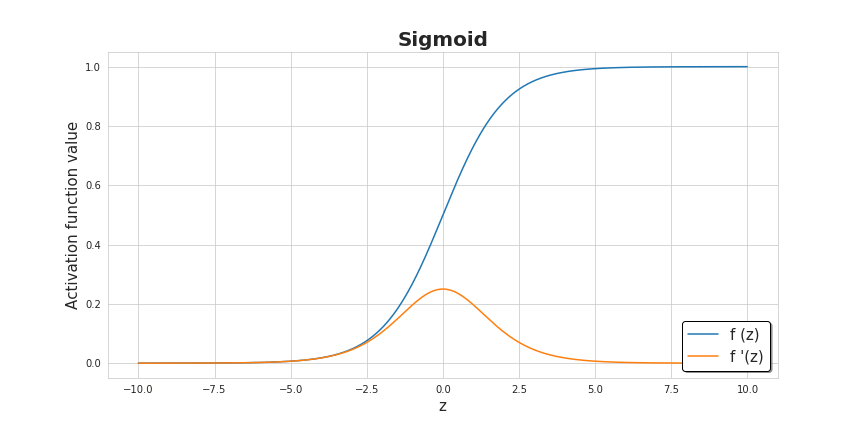Figure 4. Activation functions used in the algorithm.

#### Activation functions

Amongst all the functions that we will use, there are a few very simple but powerful ones. Activation functions can be written in a single line of code, but they give the neural nets non-linearity and expressiveness that they need. “Without them, our neural network would become a combination of linear functions, so it would be just a linear function itself.” There are many activation functions, but in this project I decided to provide the possibility of using two of them — sigmoid and ReLU. To be able to go full circle and pass both forward and backward propagation, we also have to prepare their derivatives.Snippet 3. ReLU and Sigmoid activation functions and their derivatives.

#### Forward propagation

The designed neural network will have a simple architecture. The information flows in one direction — it is delivered in the form of an X matrix, and then travels through hidden units, resulting in the vector of predictions Y_hat. To make it easier to read, I split forward propagation into two separate functions — step forward for a single layer and step forward for the entire NN.Snippet 4. Single layer forward propagation step

This part of the code is probably the most straightforward and easy to understand . Given input signal from the previous layer, we compute affine transformation Z and then apply selected activation function. By using NumPy, we can leverage vectorization — performing matrix operations, for the whole layer and whole batch of examples at once. This eliminates iteration and significantly speeds up our calculations. In addition to the calculated matrix A, our function also returns an intermediate value Z. What for? The answer is shown in Figure 2. We will need Z during the backward step.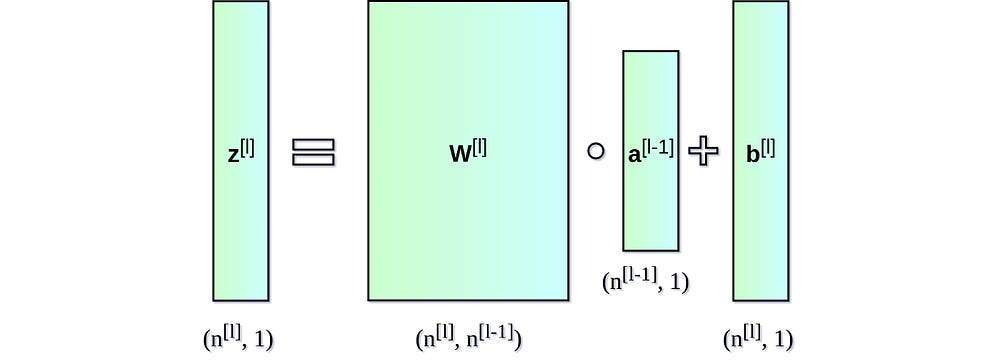Figure 5. Dimensions of individual matrices used in a forward step.

Using a pre-prepared one layer step forward function, we can now easily build a whole forward propagation step. This is a slightly more complex function, whose role is not only to perform predictions but also to organize the collection of intermediate values. It returns, among other things, Python dictionary, which contains A and Z values computed for particular layers.Snippet 5. Full forward propagation step

#### Loss function

In order to monitor our progress and make sure that we are moving in right direction, we should routinely calculate the value of the loss function. “Generally speaking, the loss function is designed to show how far we are from the ‘ideal’ solution.” It is selected according to the problem we plan to solve, and frameworks such as Keras have many options to choose from. Because I am planning to test our NN for the classification of points between two classes, I decided to use binary crossentropy, which is defined by the following formulas. To get more information about the learning process, I have also decided to implement a function that will calculate accuracy.Snippet 6. Calculating the value of the cost function and accuracy

#### Backward propagation

Sadly, backward propagation is regarded by many inexperienced deep learning enthusiasts as algorithm that is intimidating and difficult to understand. The combination of differential calculus and linear algebra very often deters people who do not have a solid mathematical training. So don’t worry too much if you don’t understand everything right away. Trust me, we all went through it.Snippet 7. Single layer backward propagation step

Often people confuse backward propaganda with gradient descent, but in fact these are two separate matters. The purpose of the first one is to calculate the gradient effectively, whereas the second one is to use the calculated gradient to optimize. In NN, we calculate the gradient of the cost function (discussed earlier) in respect to parameters, but backpropagation can be used to calculate derivatives of any function. The essence of this algorithm is the recursive use of a chain rule known from differential calculus — calculate a derivative of functions created by assembling other functions, whose derivatives we already know. This process – for one network layer – is described by the following formulas. Unfortunately, due to the fact that this article focuses mainly on practical implementation, I’ll omit the derivation. Looking at the formulas, it becomes obvious why we decided to remember the values of the A and Z matrices for intermediate layers in a forward step.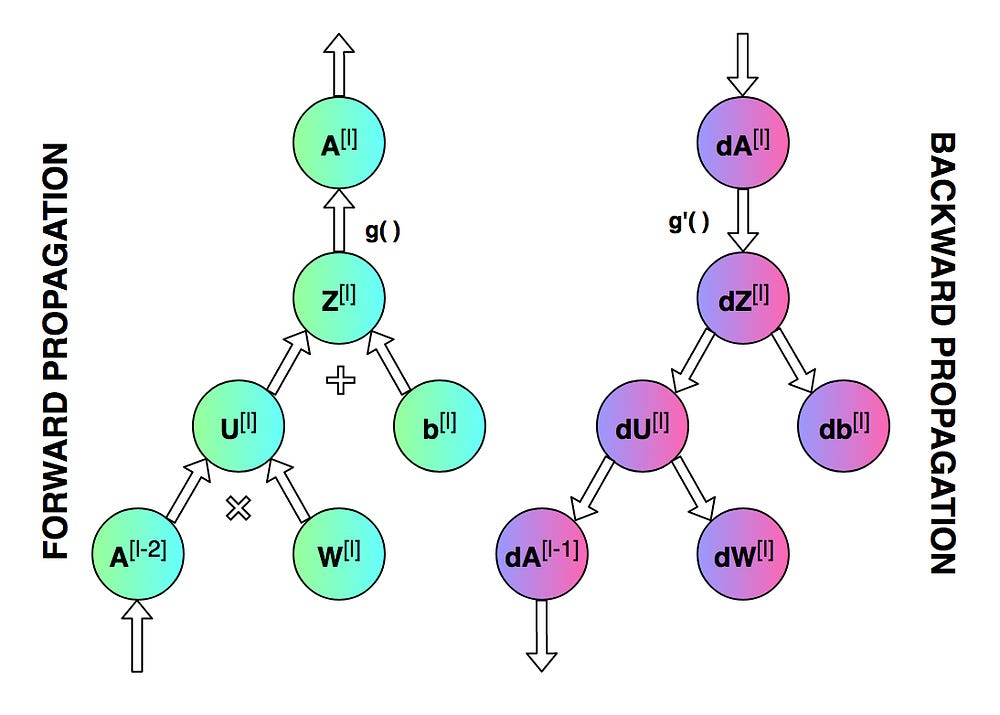Figure 6. Forward and backward propagation for a single layer.

Just like in the case of forward propagation, I decided to split the calculations into two separate functions. The first one — shown in Snippet 7 — focuses on a single layer and boils down to rewriting above formulas in NumPy. The second one, representing full backward propagation, deals primarily with key juggling to read and update values in three dictionaries. We start by calculating a derivative of the cost function with respect to the prediction vector — result of forward propagation. This is quite trivial as it only consists of rewriting the following formula. Then iterate through the layers of the network starting from the end and calculate the derivatives with respect to all parameters according to the diagram shown in Figure 6. Ultimately, function returns a python dictionary containing the gradient we are looking for.Snippet 8. Full backward propagation step

#### Updating parameters values

The goal of this method is to update network parameters using gradient optimisation. In this way, we try to bring our target function closer to a minimum. To accomplish this task, we will use two dictionaries provided as function arguments: `params_values`, which stores the current values of parameters, and `grads_values`, which stores cost function derivatives calculated with respect to these parameters. Now you only need to apply the following equations for each layer. This is a very simple optimization algorithm, but I decided to use it because it is a great starting point for more advanced optimizers, which will probably be the subject of one of my next articles.Snippet 9. Updating parameters values using gradient descent

#### Putting things together

We are finally ready. The most difficult part of the task is behind us— we have prepared all the necessary functions and now we just need to put them together in the correct order. To better understand the sequence of operations, it is worth looking again at the diagram in Figure 2. The function returns optimized weights obtained as a result of the training and the history of the metrics change during the training. In order to make a prediction, you only need to run a full forward propagation using the received weight matrix and a set of test data.Snippet 10. Training a model

#### David vs Goliath

It’s high time to see if our model can solve a simple classification problem. I generated a dataset consisting of points belonging to two classes, as shown in Figure 7. Let’s try to teach our model to classify points belonging to this distribution. For comparison, I also prepared a model in a high-level framework — Keras. Both models have the same architecture and learning rate. Still, this is a really uneven fight, as the implementation that we have prepared is probably the simplest possible one. Ultimately, both the NumPy and Keras model achieved similar accuracy of 95% on the test set. However, it took several dozen times longer for our model to reach such a result. In my opinion, this state has been caused primarily by a lack of appropriate optimisation.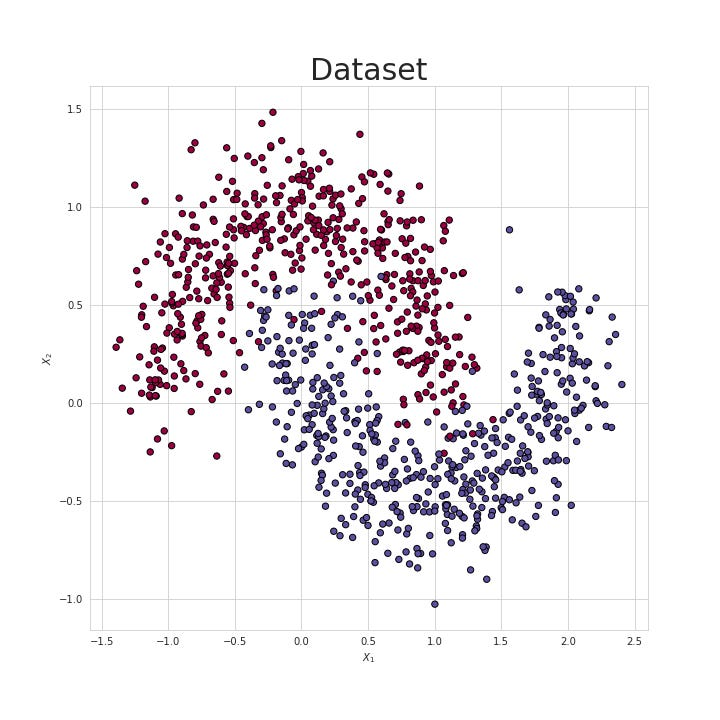Figure 7. Test dataset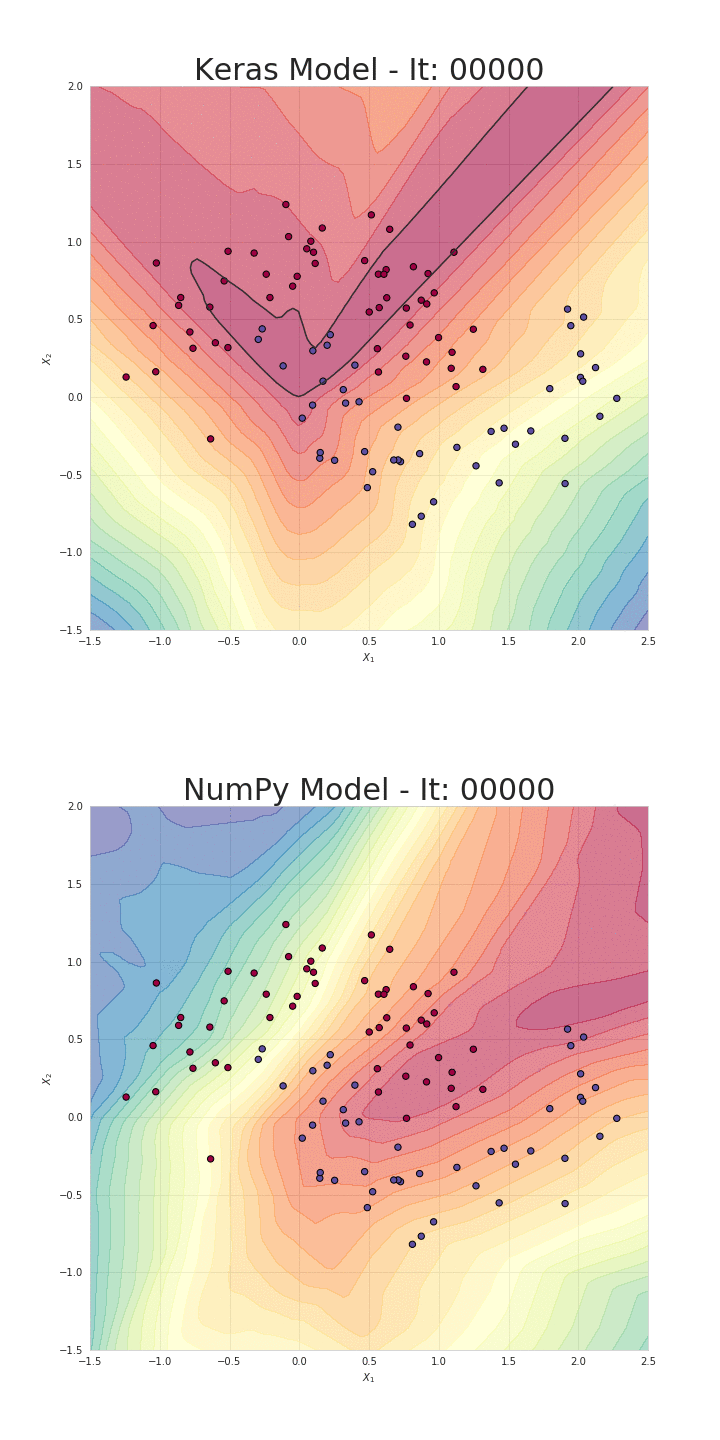Figure 8. Visualisation of the classification boundaries achieved with both models

#### Goodbye

I hope that my article has broadened your horizons and increased your understanding of compiled operations taking place inside the neural network. This would be the best reward for the effort I put into creating this post. I admit that I really learned a lot by preparing both code and comments. It is true that nothing teaches as much as getting your hands dirty.

If you have any questions or if you found an error in the source code, please let me know in the comment. And if you like this article follow me on Twitter and Medium and see other projects I’m working on, on GitHub and Kaggle. This article is the third part of the “Mysteries of Neural Networks” series, if you haven’t had the opportunity yet, read the other articles. Stay curious!

Source: Deep Learning on Medium